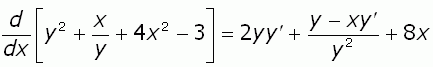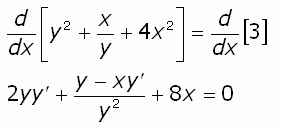SEARCH HOMEMath Central Quandaries & QueriesQuestion from Charles, a student: I need help computing y' by implicit differentiation the question is: y^2 + x/y + 4x^2 - 3Hi Charles.

With implicit differentiation, you just have to remember that y is a function of x, so if the derivative of y is y′, then the derivative of y2 is 2y y′ (I used the chain rule).

So the derivative of y2 + x/y + 4x2 - 3 (with respect to x, which is what we usually use) is:Remember that y′ is quite distinct from y, so when you simplify it you should be careful not to combine them improperly.

To actually finish the question, you generally need to solve for y′, but you didn't give us an equal sign to finish the problem (unless perhaps the - 3 should have been = 3). If you meant "equals" 3, then the equation becomesand you just solve for y′ as in any normal equation.

Hope this helps,
Stephen La Rocque.Math Central is supported by the University of Regina and The Pacific Institute for the Mathematical Sciences.